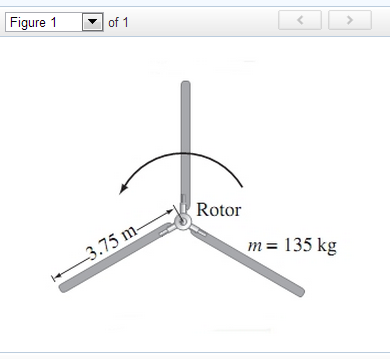# A.)  If each of the three rotor helicopter blades is 3.75 m long and has a mass...

###### Question:

A.)  If each of the three rotor helicopter blades is 3.75 m long and has a mass of 135 kg, calculate the moment of inertia of the three rotor blades about the axis of rotation.

B.)How much torque must the motor apply to bring the blades from rest up to a speed of 4.1

rev/s in 7.6s ?If each of the three rotor helicopter blades is 3.75 m long and has a mass of 135 kg, calculate the moment of inertia of the three rotor blades about the axis of rotation. How much torque must the motor apply to bring the blades from rest up to a speed of 4.1 rev/s in 7.6s ?

#### Similar Solved Questions

##### Ravait Corporation uses the weighted-average method in its process costing system. The Molding Department is the...
Ravait Corporation uses the weighted-average method in its process costing system. The Molding Department is the second department in its production process The data below summarize thedepartment's operations in January Percent Complete with Respect to Conversion 86 % Units Beginning work in pro...
##### М. Table 1: _58cm (20 points) Object Image Magnification Distance Distance p(cm) q(cm) 200 80 190...
М. Table 1: _58cm (20 points) Object Image Magnification Distance Distance p(cm) q(cm) 200 80 190 82 180 84 170 86 160 89.0 150 92 140 96 130 102 120 109 110 119.8 100 134 90 156 Complete Table 1 by calculating the magnifications for each of the object distances. Show work for one of these cal...